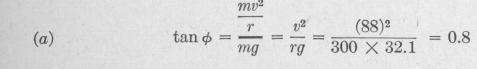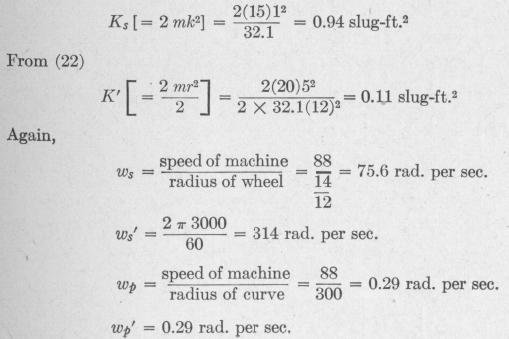Previous Page Index(For best results view this page as a PDF) Next Page

56   MOTION OF A SPINNING BODY

ing in either direction, there will be a torque when the ship moves in a curved course.

In the case of a locomotive rounding a curve, the change in direction of the axles of the drive wheels develops a torque tending to lift the drivers from the inside rail. When a side-wheel steamer turns there is a similar torque. When a rapidly moving motorcycle makes a sharp turn, the axle of the flywheel develops such a large gyroscopic couple that the rider is compelled to lean much more toward the inside of the curve than he would if the machine had no flywheel.

It should be kept in mind that (55) and all equations derived from it apply only to bodies that are symmetrical with respect to the spin-axis, that is, to bodies for which the moments of inertia about all axes through, and perpendicular to, the spin-axis are equal. It applies to rifled projectiles, electric generator and motor armatures, pulleys, flywheels and turbine runners.

This equation requires modification to make it applicable to an unsymmetrical body, that is, one for which the moments of inertia about all axes through, and perpendicular to, the spinaxis are not equal. For such a body another term must be added to (55) of the form (K, - K2)wswp where K, and K2 represent the moments of inertia with respect to two perpendicular axes that intersect the spin-axis at right angles. In the case of an airplane propeller of two blades, for example, the torque L is not uniform throughout a revolution. A scheme to prevent vibration of the machine being developed by this variable torque consists in connecting the hub of the propeller to a fork on the end of the engine shaft in such a manner that the hub is free to rock about a pin perpendicular to the shaft and inclined to the long axis of the propeller at an angle of about 45 degrees.*

The unique precessional velocity given by (57) is for the case in which the precessional axis is perpendicular to the spin-axis. It can be shown that in the case of a top spinning about an axis inclined to the vertical, there are two possible values of the preces

sional velocity, depending upon the magnitude of the spin-velocity.

When the spin-velocity exceeds a certain value, the rapid preces

sion is produced. Under this condition an increase of the external

torque applied to the spinning body results in a diminution of the

precessional velocity.t

* U. S. Patent. Messick, No. 1491997, 1924. fi Deimel, Mechanics of the Gyroscope, p. 89,

MOTION OF A SPINNING BODY   57

Problem. A certain motorcycle weighing 300 lb. has two wheels each of 15 lb., radius of gyration 12 in., and outside diameter 28 in. It has two diskshaped flywheels each of 20 lb. and diameter 10 in. rotating with the same angular velocity as the wheels. The engine makes 3000 r.p.m. when the

machine is traveling 60 mi. per hr. The rider weighs 170 lb. The center of mass of the machine is 16 in. above the ground and that of the rider is 36 in. above the ground.

Find the angle through which the machine is tilted from the vertical when the machine has a speed of 60 mi. per hr. around a curve of 300-ft. radius due (a) to centripetal effect alone; (b) to gyroscopic effect alone.

Solution. 60 mi. per hr. = 88 ft. per sec.

From Fig. 45,

Therefore d = 38° 40' inclination due to centripetal effect.

(b) The inclination 0 due to gyroscopic effect is given by the equation

gyroscopic force acting horizontally at the center of mass of the system tan 0 =   weight of the system

gyroscopic torque

_ height of center of mass above ground weight of the system

The gyroscopic torque is given by the equation

L = Kswswp +K3'ws'wp'

where the unprimed quantities refer to the motorcycle wheels and the primed quantities refer to the flywheels. From (21)Previous Page Index(For best results view this page as a PDF) Next Page